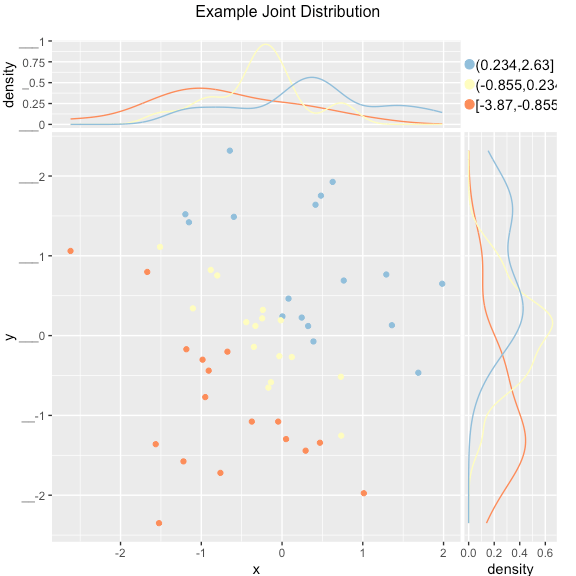# Coming up: principal components analysis

I’ve been editing a two-part three-part series Nina Zumel is writing on some of the pitfalls of improperly applied principal components analysis/regression and how to avoid them (we are using the plural spelling as used in following Everitt The Cambridge Dictionary of Statistics). The series is looking absolutely fantastic and I think it will really help people understand, properly use, and even teach the concepts.

The series includes fully worked graphical examples in R and is why we added the `ScatterHistN` plot to WVPlots (plot shown below, explained in the upcoming series).Frankly the material would have worked great as an additional chapter for Practical Data Science with R (but instead everybody is going to get it for free).

Please watch here for the series.
The complete series is now up:### jmount

Data Scientist and trainer at Win Vector LLC. One of the authors of Practical Data Science with R.

### 2 replies ›

1.daniel says:

I licked in your book and downloaded free chapter 8, in page 40 about clustering and cosine similarity you say the cosine is between 0 and 90 degrees, the correct range is 0 to 180 degrees, a small nickpicking but your readers are keen of correctness.

1.John Mount says:

Readers definitely deserve a good book. And I appreciate it looks mean to attempt to “correct an attempted correction.” But I’d like to try to explain and clarify.

But I really do not think the book said what you have repeated back. The book said assuming bounded angles, not that angles are bounded (and text analysis is a common analysis domain with reason to assume bounded angles, which I do wish we had had space to expand on).

We are happy to take criticism and maintain a free errata page for our readers (in addition to distributing free chapters, all code and data, and even some videos). I am replying to your comment here in as this is one of the few places where we can try to correct such mis-understandings. Please don’t take this as bullying on my part. I’d really like to make to friends on this issue. Perhaps this could have been clearer in the book and I apologize for any trouble this has caused- but here is what I find when I search in the book.

Chapter 8 is available as a free download and is 40 pages long- so there is no discussion of cosine on page 40 (free sample Chapter 8 available here https://www.manning.com/books/practical-data-science-with-r ). In the book chapter 8 is pages 202 through 237 and the word “cosine” only appears on pages 203, 205, 261 264, 265, 405, 406, 407, and 415. Page 205 has the description most relevant:

```COSINE SIMILARITY

Cosine similarity is a common similarity metric in text analysis. It measures the smallest angle between two vectors (the angle theta between two vectors is assumed to be between 0 and 90 degrees). Two perpendicular vectors (theta = 90 degrees) are the most dissimilar; the cosine of 90 degrees is 0. Two parallel vectors are the most similar (identical, if you assume they’re both based at the origin); the cosine of 0 degrees is 1. From elementary geometry, you can derive that the cosine of the angle between two vectors is given by the normalized dot product between the two vectors:
dot(x, y) <- sum( x*y + x*y + ... )
cossim(x, y) <- dot(x, y)/(sqrt(dot(x,x)*dot(y,y)))
You can turn the cosine similarity into a pseudo distance by subtracting it from 1.0 (though to get an actual metric, you should use 1 - 2*acos(cossim(x,y))/pi).
```

The above was stated in the context of a “text analysis” example where the input vectors are commonly collections of indicator, frequencies, co-occurances, and rates and thus non-negative. This is why the section explicitly assumes the angle between vectors is therefore bounded between 0 and 90 degrees and the stated conversion “1 – 2*acos(cossim(x,y))/pi)” is the one used. Yes in a general signed context one would instead use the conversion “1 – acos(cossim(x,y))/pi)” (see https://en.wikipedia.org/wiki/Cosine_similarity).

So the correction I would make is to: emphasize the assumed non-negative nature of the text similarity of the assumed text problem. The stated conversion is in the one often used in the text domain, so it is in fact worth bringing up. I will in fact add some clarification to errata ( http://winvector.github.io/PDSwR/PracticalDataScienceWithRErrata.html ).

Sorry that came out long- it is really hard to be understandable, correct, concise, and set context at the same time.